Zhou, A., Bian, Y., Shen, Y., Huang, D., and Zhou, M. (2018). "Inelastic bending performances of laminated bamboo beams: Experimental investigation and analytical study," BioRes. 13(1), 131-146.

#### Abstract

Laminated bamboo (LB) is a processed bamboo-based composite fabricated by gluing bamboo strips under controlled temperature and pressure. It has many superior mechanical properties compared to commonly used wood products and is well suited for use as a construction material. The present work consisted of two parts. The first part aimed at studying the bending performances of LB beams. The stress-strain relationship of the LB composite had approximately perfect elasticity under tension, yet exhibited more complicated behavior under compression (i.e., linearity in the prior-proportional limit and nonlinearity in the post-proportional limit). The strength in tension was significantly higher than that during compression. Damage of LB beam began with the fiber yielding in the compressive zone until failure occurred when the fibers at the outermost part of the tensile zone broke. Hence, LB beams always underwent a long nonlinear process before failure. An empirical stress-strain relationship was proposed on the basis of a bilinear model. In the second part of the study, an analytical model for calculating the load-carrying capacity and deflection of LB beams was developed. Experimental results confirmed that the model had enough accuracy for design calculation.

Inelastic Bending Performances of Laminated Bamboo Beams: Experimental Investigation and Analytical Study

Aiping Zhou,a,b,* Yulin Bian,c Yurong Shen,a,b Dongsheng Huang,a,b and Mengjie Zhou d

Laminated bamboo (LB) is a processed bamboo-based composite fabricated by gluing bamboo strips under controlled temperature and pressure. It has many superior mechanical properties compared to commonly used wood products and is well suited for use as a construction material. The present work consisted of two parts. The first part aimed at studying the bending performances of LB beams. The stress-strain relationship of the LB composite had approximately perfect elasticity under tension, yet exhibited more complicated behavior under compression (i.e., linearity in the prior-proportional limit and nonlinearity in the post-proportional limit). The strength in tension was significantly higher than that during compression. Damage of LB beam began with the fiber yielding in the compressive zone until failure occurred when the fibers at the outermost part of the tensile zone broke. Hence, LB beams always underwent a long nonlinear process before failure. An empirical stress-strain relationship was proposed on the basis of a bilinear model. In the second part of the study, an analytical model for calculating the load-carrying capacity and deflection of LB beams was developed. Experimental results confirmed that the model had enough accuracy for design calculation.

Keywords: Laminated bamboo; Bamboo and wood composites; Stress-strain relationship; Inelastic analysis; Bamboo beam

Contact information: a: School of Civil Engineering, Nanjing Forestry University, 159#, Longpan Road, Nanjing,210037,China; b: Jiangsu Co-Innovative Center for Efficient Utilization of Forestry Resources, 159#, Longpan Road, Nanjing,210037,China; c: Wuxi Institute of Commerce, 809#, Qianhu Road,Wuxi, 214153, China; d: School of Architecture, Nanjing TECH University, 30#, Puzhu Road, Nanjing, 211800,China; *Corresponding author: zaping2007@163.com

INTRODUCTION

Laminated bamboo (LB) is a processed bamboo-based composite manufactured with bamboo strips (Li et al. 2015; Huang et al. 2016). Four- to 5-year-old Phyllostachys bamboo culms with a 100-mm cross-section diameter (a common bamboo species grown in southwest China) are typically used to manufacture LB composites (Fig. 1a). Culms are further cut into strips that are approximately 3 m in length, 18 mm in width, and 2 mm in thickness. A machine removes the outermost and innermost parts of bamboo strips (Fig. 1b). The outermost part of bamboo is often full of wax, which causes difficulty in gluing. The innermost part of bamboo contains little fiber and as such has very low strength. This can severely weaken the adhesion strength between two strips. Thus, the outermost and innermost parts must be removed. The strips are oven-dried at approximately 60 °C until the moisture content is less than 11% (Fig. 1c), and the strips are sprayed with phenolic resin (Fig. 1d). The phenolic treated strips are arranged parallel to form a blank flat surface (Fig. 1e). The dimensions of the blank flat are decided according to manufacturing purposes, and the length can be longer than that of the strips using a special joint technique. Blank flats are placed in a machine to make LB panels under controlled temperature and pressure until the resin solidifies at the surface (Fig. 1f). Figure 1g shows one of the LB composite end products, and the composite can be made into different dimensions to satisfy diverse requirements of structural members. The blank flats can be made into a LB panel. The LB columns or beams can be obtained by further gluing together a LB panel in prescribed dimensions. Because the strips from the different parts of bamboo culms are arranged parallel in a longitudinal direction and are uniformly arranged in a transverse direction, the gradient of the mechanical properties in the transverse direction of original bamboo disappears in a macro sense. Hence, the LB composite may be treated as a transversely isotropic material.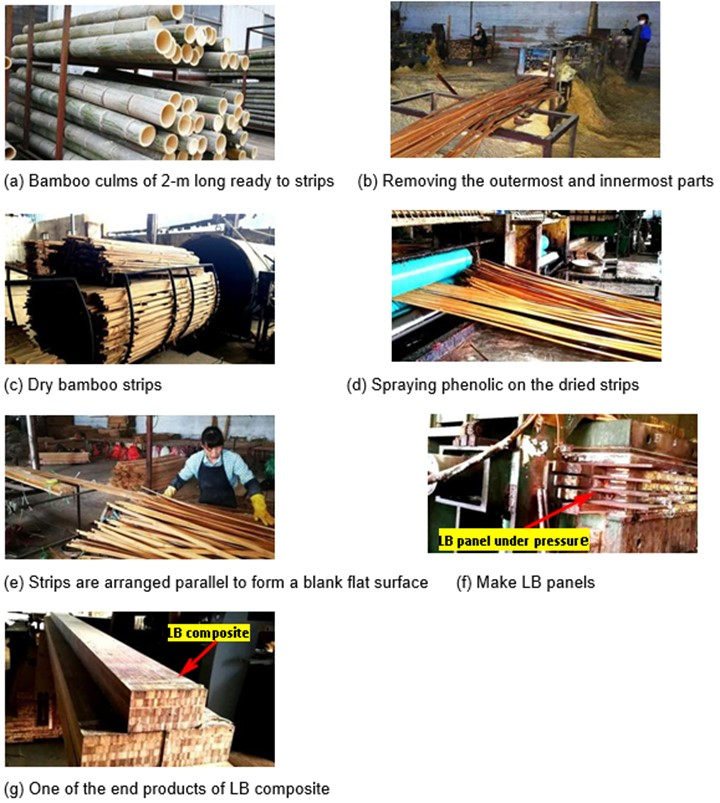Fig. 1. The brief manufacturing processes of a LB composite

Bamboo is a wood-like material because wood and bamboo have a similar microstructure (Amada et al. 1997; US Department of Agriculture 2010). Because of its excellent mechanical performance, LB composite is an attractive alternative for traditional building materials and well suited for use as decks, beams, and columns. More recently, Li et al. (2015) studied flexural performance of LB beams, and proposed a calculation formula for the ultimate bending moment. The formula involved strains of materials, which is not suitable for design calculation. Huang et al. (2016) invented a hollow deck made with LB composite. An experimental study indicated that the strength of an LB hollow deck is much higher than that of a concrete deck with the same dimensions. The tensile constitutive law exhibited perfectly elastic behaviors, whereas the compressive constitutive law presented distinct nonlinearity once the stress exceeds the proportional limit. By considering the compressive nonlinearity of a LB composite, Huang et al.(2016) developed an inelastic model for the ultimate-state analysis of LB hollow decks.

Many solid wood composites, wood-based composites, and bamboo-based composites present similar constitutive relationships in the parallel-to-grain direction, i.e. they all exhibit nearly perfect-linearity in tension and remarkable nonlinearities in compression (Moses and Prion 2004; Galicki and Czech 2005; Zhou et al. 2012; Huang et al. 2013; Li et al. 2015; Huang et al. 2015a). For this reason, the current bamboo design method follows the design philosophy of wood structures. Although the nonlinear behavior of wood products has been considered for several decades (Ramos 1961; Booth 1964), it is always ignored in the design calculation of wood structures (Canadian Wood Council 2010; US Department of Agriculture 2010; ANSI /AF&PA NDS 2012). Current wood design codes around the world recommend formulas for load-carrying capacity calculation that are all linearly-based, although they state that the ultimate-state-based philosophy should be involved in the building structure design. For LB bending members, serious errors may occur if load-carrying capacities are evaluated according to current wood design codes (US Department of Agriculture 2010). Hence, it is essential to estimate the load-carrying capacity of LB elements by taking the constitutive nonlinearity into consideration. For the sake of developing an analytical model for design calculations, the present work proposes an empirical stress-strain relationship of LB composite based on the bilinear constitutive law. An analytical model based on a bilinear stress-strain relationship was developed to estimate the load-carrying capacity of the LB bending element.

EXPERIMENTAL

Materials

Materials for test mechanical properties of LB composites

Five-year-old bamboo culms were selected (Xingda Bamboo Industry Co. Ltd., Shaowu, Fujian Province, China) to fabricate the LB composites for experiments. Test specimens were designed in accordance with ASTM D143-14 (2014). Figure 2 schematically illustrates the configuration of tensile specimens, and 200-mm-long prisms of 50 mm × 50 mm rectangular cross-sections were used as compressive samples.

Materials for testing bending performance of LB beams

In total, 10 LB beams were tested. All of the test samples had the same dimensions of 2100-mm-long, 80-mm-wide, and 160-mm-thick. The LB beams were manufactured by Xingda Bamboo Industry Co., Ltd., in accordance with Fig. 1.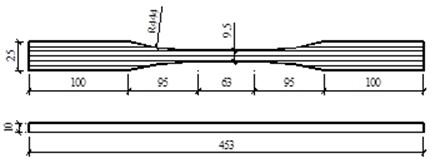Fig. 2. Dimensions of tensile specimens (mm)

Methods

Methods for mechanical properties of LB composites

Test (according to ASTM D 143-14 (2014)) loads were applied to the specimens by the movable servo actuator of the test machine. Loading was controlled by the moving speed of the actuator at a rate of 0.5 mm/min for the tensile test and 2 mm/min for the compressive test. Both the longitudinal and transverse strains at the middle of each specimen were measured using strain gauges. The values of the loads and strains were simultaneously recorded at the frequency of 1 Hz with a TDS-530 data logger (TML, Sokki Kenkyujo, Tokyo, Japan) data acquisition instrument. Young’s modulus, Poisson’s ratio, and strength were respectively evaluated as follows,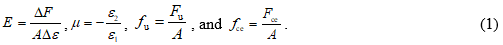where E is Young’s modulus (N/mm2), F is the load increment (N), is the strain increment (%) corresponding to Fis Poisson’s ratio, 1 and 2 are the longitudinal and transverse strains (%), respectively, A stands for the sectional area of the test samples (mm2), and Fu and Fce denote the ultimate load and compressive load associated with the proportional limit (N), respectively. The parameters fu and fce are the ultimate strength and compressive strength associated with the proportional limit (MPa), respectively. It should be emphasized that only the data chosen from linear responses are valid for evaluating elastic properties. The Young’s modulus (E) is actually determined by taking the slope of the line fitted to the experimental points.

Testing bending performance of LB beams

To understand the behavior of LB bending components, 4-point bending experiments for simply supported LB beams were conducted according to ASTM D 198-15 (2015). The objective of the tests was to investigate the failure modes and failure mechanisms of LB beams. The test setup is schematically illustrated in Fig. 3, and the configurations of test samples, test span, and the length of shear span are also reported in the figure. Five strain gauges were uniformly and longitudinally glued over the side surface mid-span to measure the strain at that particular point. A laser deformation sensor was installed under the mid-span to monitor and measure the deflection of the test beams. Test load was symmetrically applied to test samples at two points equidistant from the reactions. Load was monotonically added and controlled via mid-span displacement at a rate of 2.5 mm/min to ensure that the specimen failed in approximately 20 min so that the primary creep of material could be neglected. The values of loading, strain, and deflection mentioned above were simultaneously recorded by a data acquisition instrument at a frequency of 1 Hz.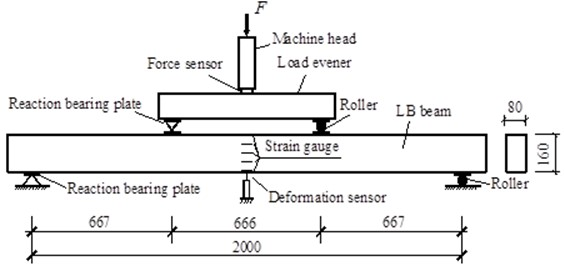Fig. 3. Test setup

RESULTS AND DISCUSSION

Stress-strain Relationship of LB

As discussed above, the LB composite can be treated as an orthotropic material on a macroscopic scale. Similar to other wood-like composites, the constitutive law and failure criteria of LB composites are too complicated to be theoretically determined. Fortunately, only the longitudinal properties are involved in the evaluation of the bending behavior of beams if Euler’s beam theory (Timoshenko 1953) is employed. Accordingly, the authors only considered the parallel-to-grain properties of LB composite in the present study. Tests determined empirical constitutive relations and associated parameters and the test results are briefly presented hereafter.

Empirical equation of stress-strain relationships

Figures 4a and 4b present the stress-strain curves of tension and compression. Nearly perfect linearity can be observed in tensile constitutive relations. However, the compressive stress-strain relationship exhibited more complicated behaviors. Approximately three segments can be observed in the compressive stress-strain curves. The first one is a linear segment starting from the beginning of the loading to the proportional limit. The second segment is a strain-softening segment starting from the proportional limit to the curve peak. The last one is the declining segment which is usually not evident; hence, it can be omitted.

Experimental data analysis demonstrates that the parallel-to-grain stress-strain relationship of the LB composite is quite similar to that of the other wood-based composites but the compressive nonlinearity is more impressive, as shown in Fig. 4b. Therefore, the ultimate load-carrying capacity and deformation would be underestimated if the nonlinearity was ignored. This is in agreement with Ramos (1961), who used a clear wood test and found that the compressive nonlinearity can affect the bending of the compression stress block. Hence, nonlinear stress-strain relationships of wood or wood-like composites have been extensively studied in past decades. To date, four main types of constitutive models are commonly accepted, as is schematically illustrated in Fig. 4c.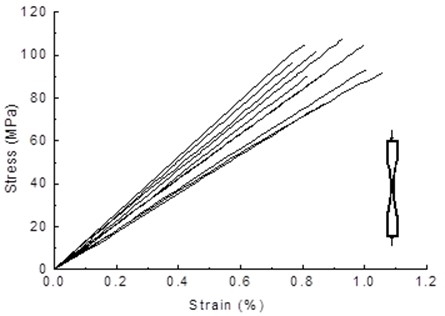1. Experimental tensile stress-strain curves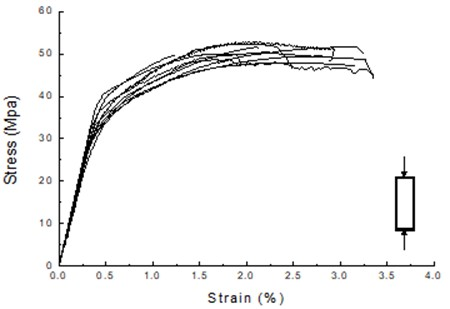1. Experimental compressive stress-strain curves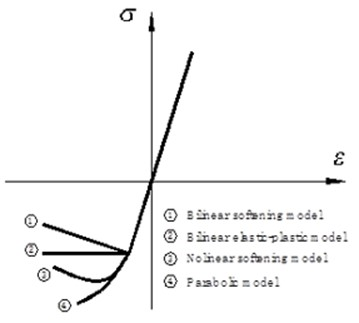1. Empirical bi-linear model

Fig. 4. Stress-strain relationships of LB composite in parallel-to-grain direction

The bilinear elastic-plastic relationship and the bilinear-softening stress-strain relationship proposed by Neely (1898) and Bazan (1980), respectively, are popular models among them. The two models take the proportional limit as the maximum compressive strength. As such, they may underestimate the load-carrying capacity of wood members. Zakić (1974) observed the nonlinear soft process of compression and proposed a parabolic model for the compressive stress-strain relationship of solid wood. Usually, the parabolic and polynomial model can provide a good approximation for solid wood in the compression parallel to grain. Huang et al. (2015a) confirmed that the nonlinearity of the parallel-to-grain stress-strain relationship of bamboo-based composites can be modeled by parabolic curves and provided an equation as follows,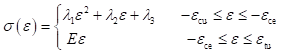(2)

where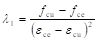,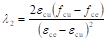,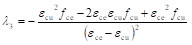,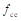and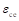,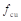and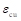are the proportional compressive limit stress and corresponding strain, ultimate compressive stress and corresponding strain, respectively. Thus,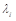(= 1, 2, 3) are material constants that can be determined by the ASTM D 143-14 (2014) standard. The above equation is inconvenient for design calculation because strain parameters are needed in Eq. 2.

To develop an applicable model for design calculation, the present study used a bilinear equation to model the compressive stress-strain of LB composite as shown in Fig. 5.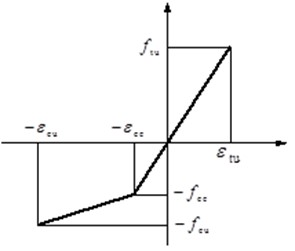Fig. 5. Commonly used parallel to grain stress-strain relationship

The empirical formula of the bilinear stress-strain model is expressed as,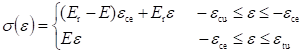(3)

where εcuεce, and εtu are ultimate compressive strain (%), proportional compressive strain (%), and ultimate tensile strain (%), respectively. The secant compressive modulus is E(N/mm2), which is calculated as follows: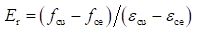(4)

The parameters of the critical point of Eq. 3 are presented in Table 1.

Table 1. Mechanical Properties of LB Composites in Parallel-to-grain Direction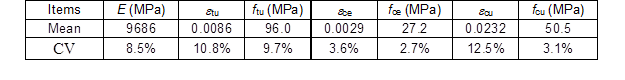In Table 1, CV represents the coefficient of variation.

Discussion for Bending Performance

Figure 6 graphically illustrates the typical failure mode of LB bending members. Due to the fact that the elastic compression limit is lower than ultimate tensile strength, damage starts and gradually develops in the compressive area once the load exceeds the proportional limit. The damage mechanisms are mainly induced by fiber buckling in compressive area and fine crack extension in bounded surface. Therefore the bending stiffness of beam was progressively degraded with the augmentation of loading. The beam was finally failed due to the breaks in the bottom layer.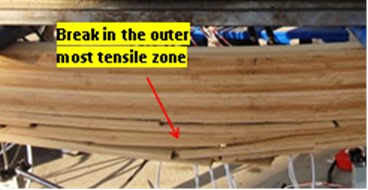Fig. 6. Typical failure mode of LB beam

The experimental load-deflection curves (gray lines) of the mid-span and the deflection calculated results discussed later (red line) are reported in Fig.7.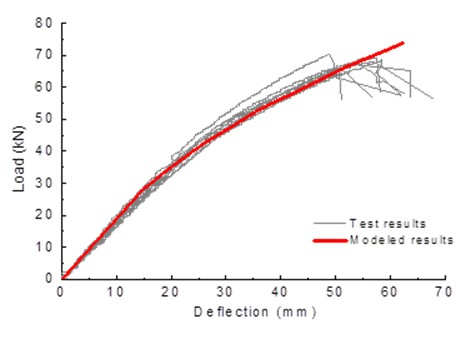Fig. 7. The load-deflection curves at mid-span obtained by test and calculation for LB beams

It was observed that the load-deformation curves exhibited distinct nonlinearity when the load exceeded the proportional limit. The load at the proportional limit was approximately half of the maximum load, and the load-displacement curve gradually deflected to the horizontal axis. During this process, the neutral axis of nonlinear cross section is continuously offsetting towards the convex side. This implied that the nonlinearity of LB beam cannot be ignored in the evaluation of load-carrying capacity. Hence, it is essential to estimate the load-carrying capacity of LB elements by taking the constitutive nonlinearity into consideration.

Figure 8 presents the strain distribution over the failure section. An approximately linear distribution of strain over depth can be observed. It also can be seen that the strain in the outmost compressive zone of the failure cross-section could be greater than 8000, which far exceeded the strain of the compressive proportional limit (ce = 2900 ), as shown in Table 1. This implied that the material was in a nonlinear state before failure.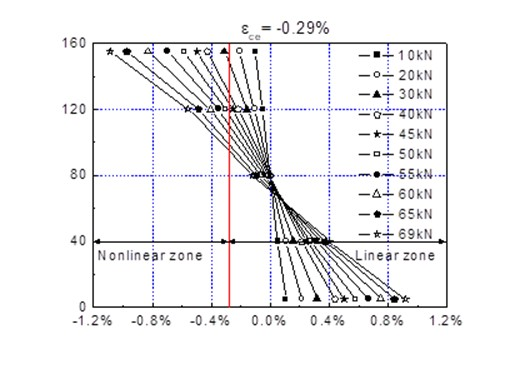Fig. 8. Variation of strain diaphragm against load augmentation

Hence, it was concluded from above that the failure of LB bending member was a process of progressive damage. Damage began with material yielding in the compressive zone and crack generating and crack expanding in the tensile zone. Failure occurred when the laminates at the bottom of the beam were broken. During the whole loading process, strains were distributed in a linear manner over the cross-section, which indicated that the plane hypothesis was valid.

Modeling for Ultimate State Calculation

To model the nonlinear process of LB bending members, the authors adopted the following assumptions based on the results of the experiments: (1) the cross-section remains plane after bending and (2) materials in tensile zone remain in a linear state and the stress at the outermost part of the tensile zone equals ftu when failure occurs.

The above assumptions implied that the stress distribution of a failure cross-section was divided into 3 zones over its depth. The top area is the plastic compressive zone (PCZ), in which the stresses exceed the compressive proportional limit (fce) and reach compressive strength (fcu) in the outmost surface. The area between the PCZ and the neutral axis is the elastic compressive zone (ECZ), in which the material works in a compressive elastic state. The stress in the boundary between the PCZ and the ECZ just reaches the proportional limit (fce). The area under the neutral axis is the elastic tensile zone (ETZ), in which the material is always working in an elastic state. Therefore, the stress and strain distribution over the damaged cross-section can be schematically illustrated, as depicted in Fig. 9.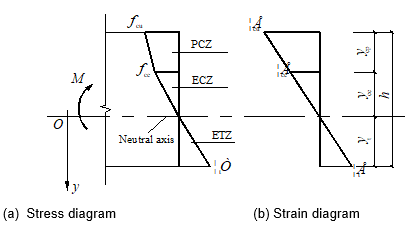Fig. 9. Diagrams of the stress and strain distributions over the moment section

For the sake of convenience, a coordinate, y, which originates from the neutral axis of bending and moves in a positive direction toward the convex side, was employed to functionally describe the stress-strain distribution. Equation 5 gives the stress distribution with respect to y over the failure cross-section,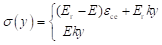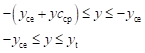(5)

where E is the longitudinal Young’s modulus of LB composite (N/mm2), k stands for the bending curvature at the critical cross section (m-1), yceycp, and yt are the depths (mm) of the ECZ, the PCZ, and the ETZ, respectively. The resultant forces of the cross-section were calculated as follows,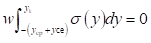(6a)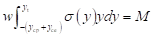(6b)

where w is the width of the LB beam (mm). By substituting Eq. 5 into Eq. 6 and considering the geometrical condition, yce + ycp + yt = h, the resultant moment in a nonlinear state and the associated depths of each zone can be obtained as follows: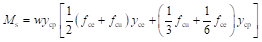(7)

The total resultant moment in the failure section can be expressed as: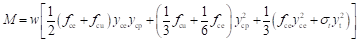(8)

The depths of the PCZ, the ECZ, and the ETZ are be calculated as: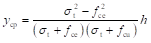(9a)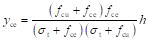(9b)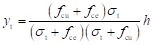(9c)

By replacing ftu for t in Eq. 9, one can obtain the depths of the PCZ, the ECZ, and the ETZ under the ultimate state, and further insert ycpyce, and yt into Eq. 8. The ultimate load-carrying capacity can be calculated as: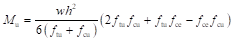(10)

It can be observed that only the strength parameters of a material and the dimensions of a section are involved in calculating the load-carrying capacity with Eq. 10. Hence, it is practical for design application. It will be validated later that Eq. 10 can achieve good agreement with the test results.

Deformation

Determining the nonlinear bending deformation of a beam is an intractable subject because the bending stiffness (EI) no longer holds the whole length of the beam once it works in a nonlinear state. The local bending curvature not only depends on the moment but also on the nonlinear development of the critical section. To calculate the ultimate deformation of beam-columns (Chen and Atsuta 1976), Huang et al. (2015b) suggested a deflection calibration method to evaluate the nonlinear deflection of beam-columns under eccentric load. The model regards beam-columns as elastic rods with plastic hinges at the critical section. Inelastic deformation is only caused by plastic hinge rotation. By assuming that the length of a plastic hinge is equal to the depth of the bending section, the ultimate deflection is obtained by calibration of the linear deflection under ultimate load. The significant advantage of this model is its applicability that allows calculating the nonlinear deflection of the bending member subjected to arbitrary loads without considering the damage process. According to the principles of the Huang et al. (2015b) method, the total deflection of a bending member consists of two parts (e and p) as shown in Fig. 10. Hence, the total deflection can be expressed as,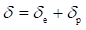(11)

where e and p are the fictitious elastic deflection (mm) and the plastic deflection (mm), respectively. The fictitious elastic deflection can be calculated by Euler’s beam theory under the objective load. The calibration item, i.e. the fictitious plastic deformation is calculated as,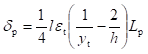(12)

where t is the tensile strain in the outermost ETZ (%) and Lp is the length of the plastic hinge (mm).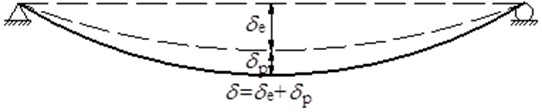Fig. 10. Deformation of the beams

Because of the complicated damage mechanism of the LB composite, it was difficult to theoretically determine the length Lp of the plastic hinge. This was revealed by experiments that showed that the nonlinear process of LB bending members was developed by microvoids coalescing and small cracks expanding. However, the fully developed nonlinear section does not truly exist.

The plastic hinge is only a fictitious component used to approximate the nonlinear behavior of bending in a macro sense. Therefore, Lp is a parameter used to correct a nonlinear response that can be empirically determined. Previous studies suggested that Lp is the depth of the failure section. Considering the tensile elastic constitutive law in Eq. 12 gave the fictitious nonlinear deflection as follows: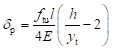(13)

For the 4-point bending specimens in this study, the ultimate deflection at the middle span was computed as: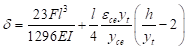(14)

where F is the force introduced by servo-actuator (N), I is the moment of inertia of critical section(mm4), and l is the span of test beam (mm).

Calculation method

The ultimate load-carrying capacity was calculated with Eqs. 8 and 9 by replacing twith ftu in each equation and the ultimate deformation was calculated with Eq. 13. Theoretically, one can trace the nonlinear processes through these equations via a step-by-step approach. However, a problem arose in practice because the stress in the outermost part of the ETZ remained unknown.

Two methods can be employed to solve this problem. One is a numerical method that involves a stationary point iteration process to determinetat each calculation step. This method is time consuming and is likely to lead to divergence due to choosing the wrong initial value for t. The other method is a progressive method, which calculates yt,i by using the stress of the outermost part of the ETZ(t,i-1) of the previous step. Provided that the increment of the loading step is small enough, the error induced by the asynchronicity of yt,i and t,i-1 is acceptable. The present work employed the second method to trace the inelastic processes of the experimental specimens. The flow chart of the calculation process is illustrated in Fig. 11.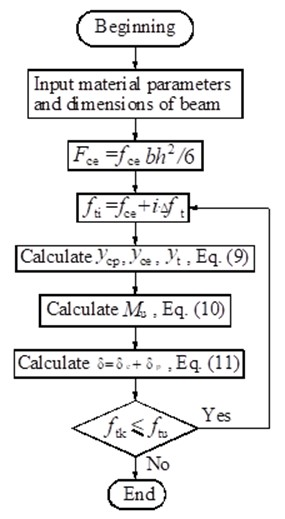Fig. 11. The flow chart of calculation

Experimental verification

To validate the method proposed above, load-carrying capacities and ultimate deflections obtained by experiments, the Huang et al. (2013) model, and the present method are compared in Table 3. The mechanical properties of parallel strand bamboo (PSB) composites in the parallel-to-grain direction are presented in Table 2.

Table 2. Mechanical Properties of PSB Composites in Parallel-to-grain Direction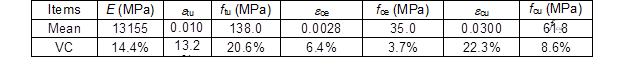The test results of LB bending samples obtained in the present work and PSB bending samples obtained by previous studies are compared. It can be concluded that the results computed by the present method closely approximate the test results. Figure 7 compares the load-deflection curves of tests conducted using the present method for LB samples. It can be observed that the calculated results fit well with the test results. The verification confirmed that the present method accurately predicts the load-carrying capacity and deflection of bamboo-based composite beams. The calibration method based on the Huang et al. (2013) model is simpler and only involves the strength parameters of materials. Although the error caused by different simulated model is higher, it is still in the acceptable range of engineering calculation. Furthermore, the calculation formula in this study eliminates the parameters of strains which usually are not provided by material suppliers. Hence the formulas in this paper are more practicable for design calculation.

Table 3. Comparison of the Results between Tests and Theoretical Calculations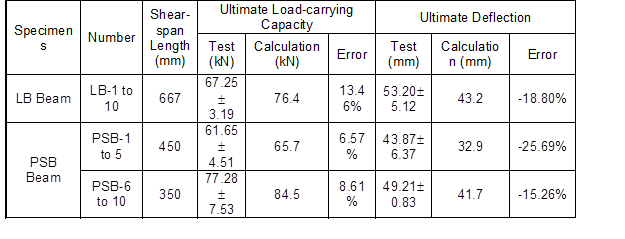CONCLUSIONS

1. The tensile stress-strain relationship was perfectly linear. The compressive stress-strain relation exhibited linear behavior prior to the proportional limit and exhibited nonlinear behavior after the proportional limit. The compressive proportional limit was only approximately half of the ultimate strength. The nonlinear segment of the compressive stress-strain relationship can be simulated by a bi-linear model.
2. Due to the lower elastic compression limit and the higher tension strength, the failure of LB bending member always underwent a progressive damage process. Damage began with material yielding in the compressive zone and cracks being generated and expanding in the tensile zone. Failure occurred when the laminates at the bottom of the beam broke. During the whole loading process, strains were distributed in a linear manner over the cross-section, which indicated that the plane hypothesis was valid.
3. The bilinear constitutive law model is suitable for the nonlinear analysis of LB bending members. Due to the fact that only strength parameters are involved in the calculation of the bilinear constitutive law, the method proposed in this study is more applicable for design-oriented calculation. Experimental validation indicated that the method was accurate enough for engineering application.

ACKNOWLEDGMENTS

This research was supported by the National Science Fund of China (No. 51578291), National Key Research Project (No. 2017YFF0207203-04), National Demonstration Project of Forestry Science and Technology and the Priority Academic Development Program of Jiangsu Higher Education Institutions.

REFERENCES CITED

Amada, S., Ichikawa, Y., Munekata, T., Nagase, Y., and Shimizu, H. (1997). “Fiber texture and mechanical graded structure of bamboo,” Composites Part B: Engineering 28(1-2), 13-20. DOI: 10.1016/S1359-8368(96)00020-0

ANSI /AF&PA NDS (2012). “National design specification for wood construction,” American Forest and Paper Association, Washington, DC.

ASTM D143-14 (2014). “Standard test methods for small clear specimens of timber,” ASTM International, West Conshohocken, PA.

ASTM D198-15 (2015). “Standard test methods of static test of lumber in structural sizes,” ASTM International, West Conshohocken, PA.

Bazan, I. M. M. (1980) Ultimate Bending Strength of Timber Beams, Ph.D. Dissertation, Nova Scotia Technology College, Halifax, Nova Scotia.

Booth, L. G. (1964). “The strength testing of timber during the 17th and 18th centuries,” Journal of the Institute of Wood Science 13, 5-30.

Chen, W. F., and Atsuta, T. (1976). Theory of Beam-columns, In-Plane Behaviour and Design, Vol. 1, McGraw-Hill, Inc., New York.

EN 1995-1-1 (2004). “Eurocode 5: Design of timber structures,” European Committee for Standardization, Brussels, Belgium.

Galicki, J., and Czech, M. (2005). “Tensile strength of softwood in LR orthotropy plane,” Mechanics of Materials 37(6), 667-686. DOI: 10.1016/j.mechmat.2004.07.001

Huang, D., Bian, Y., Zhou, A., and Sheng, B. (2015a). “Experimental study on stress-strain relationships and failure mechanisms of parallel strand bamboo made from phyllostachys,” Construction and Building Materials 2015(77), 130-138. DOI: 10.1016/j.conbuildmat.2014.12.012

Huang, D., Bian, Y., Huang, D., Zhou, A., and Sheng, B. (2015b). “An ultimate-based-model for inelastic analysis of intermediate slenderness PSB columns under eccentrically compressive load,” Construction and Building Materials 94, 306-314. DOI: 10.1016/j.conbuildmat.2015.06.059

Huang, D., Zhou, A., and Bian, Y. (2013). “Experimental and analytical study on the nonlinear bending of parallel strand bamboo beams,” Construction and Building Materials 35(3), 585-592. DOI: 10.1016/j.conbuildmat.2013.03.050

Huang, Z., Chen, Z., Huang, D., and Zhou, A. (2016). “The ultimate load-carrying capacity and deformation of laminated bamboo hollow decks: Experimental investigation and inelastic analysis,” Construction and Building Materials 2016(117), 190-197. DOI: 10.1016/j.conbuildmat.2016.04.115

Li, H., Deeks, A. J., Zhang, Q., and Wu, G. (2015). “Flexural performance of laminated bamboo lumber beams,” BioResources 11(1), 929-943. DOI: 10.15376/biores.11.1.929-943

Moses, D. M., and Prion, H. G. L. (2004). “Stress and failure analysis of wood composite: A new model,” Composites Part B: Engineering 35(3), 251-261. DOI: 10.1016/j.compositesb.2003.10.002

Neely, S. T. (1898). “Relation of compression-endwise to breaking load of beam,” in: Progress in Timber Physics, USDA Forest Service, Washington D.C., pp. 13-17.

Ramos, A. N. (1961). Stress-strain Distribution in Douglas-fir Beams within the Plastic Range (Report No. 2231), U.S. Department of Agriculture Forest Products Laboratory, Madison, WI.

Timoshenko, S. P. (1953). History of Strength of Materials, McGraw Hill, New York, United States.

United States Department of Agriculture (2010). Wood HandbookWood as an Engineering Material (Centennial Edition), Forest Products Laboratory, Madison, WI.

Zakić, B. D. (1974). “Inelastic bending of wood beams,” Journal of the Structural Division99(st10), 2079-2092.

Zhou, A., Huang, D., Li, H., and Su, Y. (2012). “Hybrid approach to determine the mechanical parameters of fibers and matrixes of bamboo,” Construction and Building Materials 35, 191-196. DOI: 10.1016/j.conbuildmat.2012.03.011

Article submitted: July 19, 2017; Peer review completed: September 23, 2017; Revised version received: November 1, 2017; Accepted: November 2, 2017; Published: November 7, 2017.

DOI: 10.15376/biores.13.1.131-146